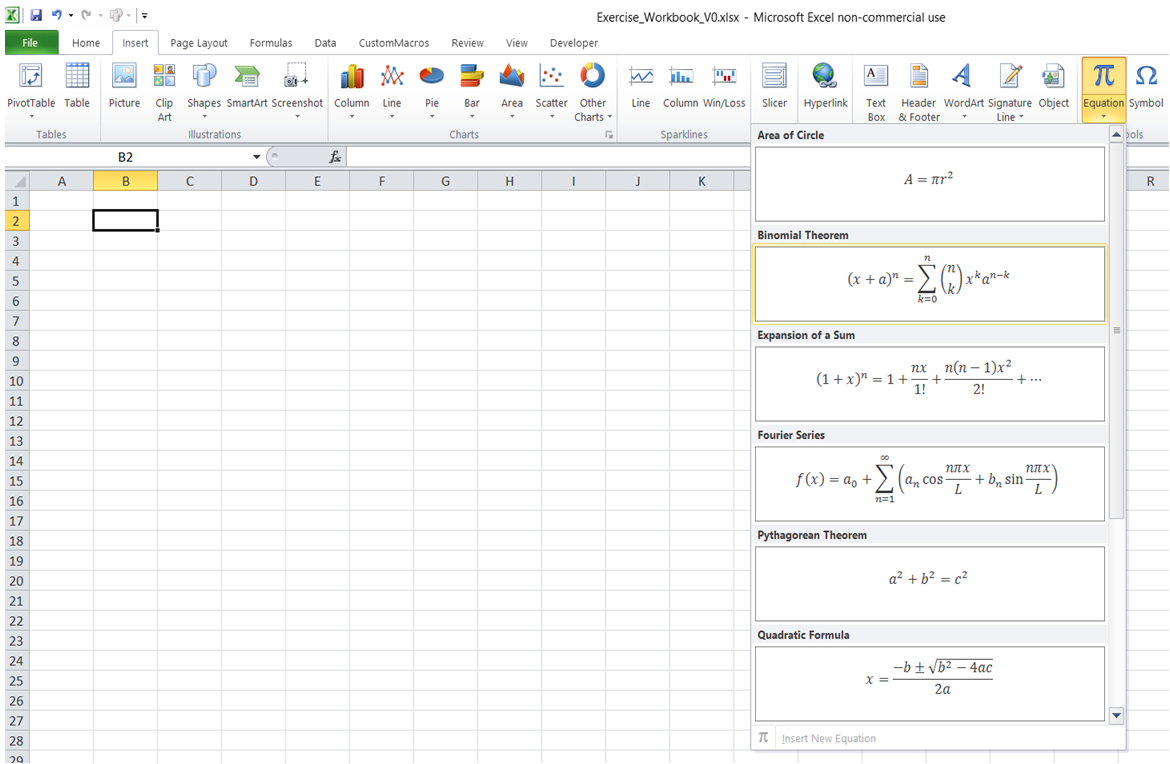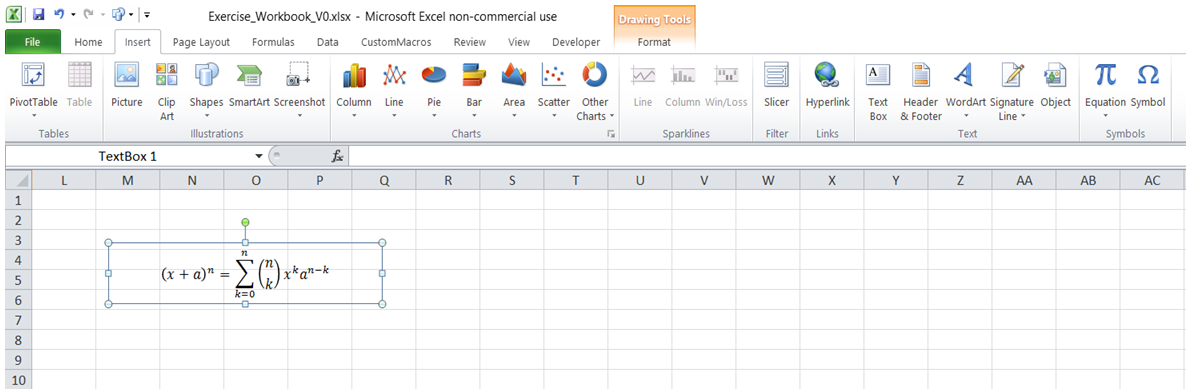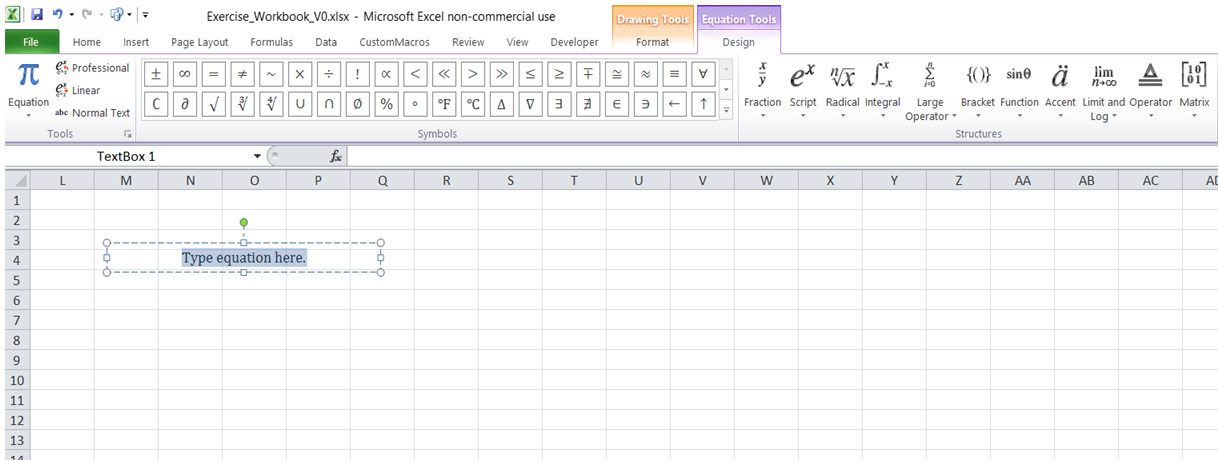## How to enter equation in Excel

To do it in Excel, here is the answer:

a) Under "Insert" tab, click on "Equation" -> "Binomial Theorm".b) The equation is displayed as below.c) To enter an equation not in standard list, insert a shape (Click on Shapes under "Insert" tab) and with shape selected click on "Equation". "Insert New Equation" (Refer first screenshot) will get activated.

A separate tab "Equation Tools" with all the available elements for generating Engineering and Mathematical formulas is diplayed. Use the elements as needed to generate the equation.